Smartick is an advanced online program that teaches kids math and coding in only 15 min. a day

Apr22

# How to Calculate the Least Common Multiple Using a 100 Square

In a previous post, we learned what a 100 square was and how to visualize and know the multiples of a number on it. If you have not read the post I would recommend that you do so before continuing, and if you don’t know what the least common multiple is, check out this post.

Today we are going to use a 100 square to understand and visualize the concept of least common multiple (LCM) and how to solve activities related to it. Keep in mind that you can only use this table if the least common multiple is less than or equal to 100.

To find the least common multiple of two numbers using a 100 square, you need to follow a series of steps. For example, if you want to know the LCM of 5 and 7:

#### 1.- Multiples of the first number

The first thing we have to do is to find the multiples of 5: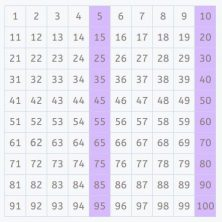#### 2.- Multiples of the second number

Now, we want to know the multiples of 7: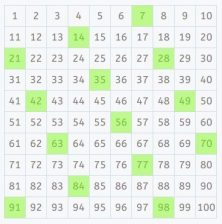#### 3.- Choose the smaller of the common multiples

Then we can see that all of the previous numbers which are common multiples of 5 and 7, and of them, the smallest is the LCM.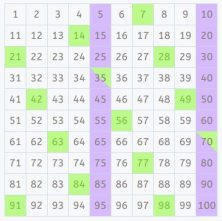In this case, the numbers 35 and 70 are multiples of 5 and 7. The smallest of these is 35 which makes 35 the LCM of 5 and 7.

### Least common multiple using a 100 square on Smartick

At Smartick we offer activities to calculate the LCM through a 100 square. For example, find the LCM of 9 and 4.

First, we indicate the multiples of 9 in the 100 square: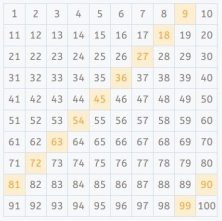Then, we point out the multiples of 4: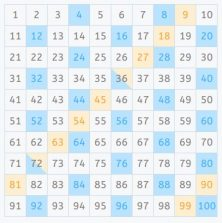We can already see that the common multiples are 36 and 72 and now select the smallest. Thus, 36 is the LCM of 9 and 4.

We have seen how easy it is to calculate the LCM with the 100 square and how simple it is to visualize and understand the concept through this tool. Now it’s your turn to practice with a square that you can download here. Try to calculate the LCM of 3 and 13, or 2 and 15.

If you want to learn more and practice with least common multiples or other contents, check out Smartick and try it for free.